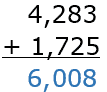## All About These 15 Worksheets

This Four Digit Addition series consists of 15 worksheets designed to help students master addition of numbers with four digits. The worksheets provide practice problems with varying levels of difficulty and formats to help students develop a strong foundation in adding four-digit numbers.

The worksheets include both vertical equations and column addition problems. Some worksheets require students to find the missing addend instead of the sum, which helps them improve their problem-solving skills and mental math abilities.

Each worksheet includes a mix of problems with and without regrouping. This helps students understand the concept of carrying over and borrowing when adding numbers with multiple digits. The worksheets also provide ample space for students to show their work and write down their answers.

Overall, these worksheets provide a comprehensive and engaging way for students to master addition of four-digit numbers. With these worksheets, students can develop a solid foundation in math and build the skills they need to succeed in higher-level math courses.

## How to Perform Four Digit Addition Problems

To perform four-digit addition problems, you should follow a step-by-step process, working from right to left (ones place to thousands place). Here’s how to do it:

1. Write the numbers – Write the numbers you want to add, aligning them vertically by their place values (ones, tens, hundreds, and thousands). Make sure the digits in each place value column line up.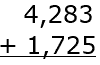2. Add the Ones Place – Start by adding the digits in the ones place (the far right column). Write the sum below the line, in the same column. If the sum is greater than 9, write down the last digit and carry over the remaining digit(s) to the next column (tens place).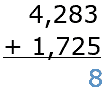3. Add the Tens Place – Add the digits in the tens place, along with any carry-over from the previous step. Write the sum below the line, in the same column. If the sum is greater than 9, write down the last digit and carry over the remaining digit(s) to the next column (hundreds place).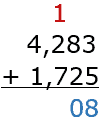4. Add the Hundreds Place – Add the digits in the hundreds place, along with any carry-over from the previous step. Write the sum below the line, in the same column. If the sum is greater than 9, write down the last digit and carry over the remaining digit(s) to the next column (thousands place).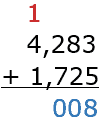5. Add the Thousands Place – Add the digits in the thousands place, along with any carry-over from the previous step. Write the sum below the line, in the same column.